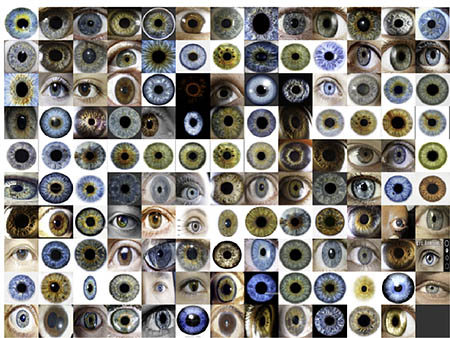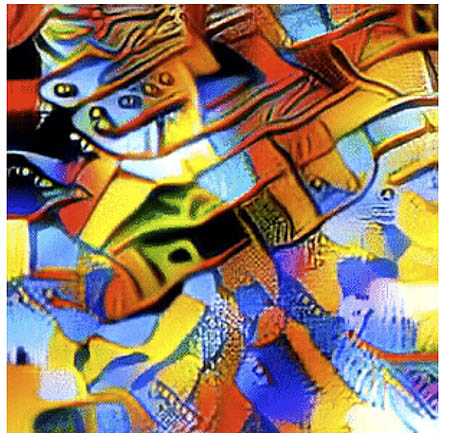# Mathematica

Also found in: Dictionary, Medical, Wikipedia.

## Mathematica

(tool, mathematics)
A popular symbolic mathematics and graphics system, developed in 1988 by Stephen Wolfram and sold by Wolfram Research. The language emphasises rules and pattern-matching. The name was suggested by Steve Jobs.

http://wri.com/mathematica/.

Stanford FTP, NCSA FTP.

Mailing list: mathgroup-request@yoda.ncsa.uiuc.edu.

Usenet newsgroup: news:comp.soft-sys.math.mathematica.

["Mathematica: A System for Doing Mathematics by Computer", Stephen Wolfram, A-W 1988].
This article is provided by FOLDOC - Free Online Dictionary of Computing (foldoc.org)

## Mathematica

Mathematical software for Windows, Mac and Linux from Wolfram Research, Inc., Champaign, IL (www.wolfram.com). Launched in 1988, Mathematica includes numerical, graphical and symbolic computation capabilities, all linked to the Wolfram functional programming language. With more than 6,000 functions, Mathematica supports 64-bit memory addressing and 64-bit long number partitioning. It also supports threading of numerical linear algebra over multiple CPUs and multicore processors. See functional programming and WolframAlpha.

Wolfram Competition
At its technology conferences, the company invites attendees to submit examples of "One-Liners," which are small amounts of Wolfram code that produce amazing results. Two of the 2018 winners follow:

``` c=Flatten@DeleteCases[WebImageSearch
["eye iris","Images",MaxItems->120],
\$Failed];
ImageCollage[ConformImages[c[[1;;Length[c]]]]]
```

The Eyes Have ItDavid DeBrota's 127 characters of Wolfram code searched the Web for "eye iris" and returned this image. (Image courtesy of Wolfram Research.)

``` i=RandomImage[1,300,ColorSpace->"RGB"];
Dynamic[i=ImageRestyle[i.i]]
```

Abstract ArtJon McLoone, Director of Wolfram's Technical Communication and Strategy produced the GIF above with 68 characters of Wolfram code. The original is actually an extremely long animated GIF that generates 50 unique frames before repeating. (Image courtesy of Wolfram Research.)
Copyright © 1981-2019 by The Computer Language Company Inc. All Rights reserved. THIS DEFINITION IS FOR PERSONAL USE ONLY. All other reproduction is strictly prohibited without permission from the publisher.
References in periodicals archive ?
The technical guide introduces the Wolfram language and Mathematica software package for representing mathematical functions, and explains the commands for input, output, differentiation, limits, integration, probability, statistics, and data analysis.
But Mathematica was conceived in a pre-internet era.
Para sus desarrolladores Wolfram Research Inc, Mathematica es un sistema para "hacer matematica por computadora".
Keywords: Computer Algebra System Mathematica and Matlab mathematical science problem.
In order to verify the mathematical model of the manipulator, describing angular displacement of 2DOF piezoelectric actuators, symbolic-numerical software Wolf ram Mathematica and solid geometry software Cabri Geometry 3D was used.
Two years ago, at Equadiff 2011 at Loughborough University, UK, when I met Francesco for the last time, I told him that Professor Constantin Corduneanu entrusted me with the task of preparing a new journal to re-launch Libertas Mathematica. Francesco was very interested, enthusiastic and eager to find out what I was going to do and our intense conversation continued via E-mail and on the phone after the Congress until the end of his life.
Content creators generate CDF documents through the Mathematica software, though the company will also offer online authoring tools as well.
Using Mathematica students were able to visualise Fourier series of functions and explore Gibbs phenomenon which is usually a part of college mathematics.
This paper provides a comparison of effectivity in the view of execution time of algorithms which ensure evolutionary-estimated programming the Turing machine implemented in Wolfram Mathematica and F# programming language using Microsoft .NET Framework.
The software packages used in this three-credit hour course that includes an extra one-hour weekly lab are Excel, Mathematica, and MATLAB, all of which have been integrated into various courses throughout our departmental curriculum.
Electrotechnical systems; calculation and analysis with Mathematica and PSpice.

Site: Follow: Share:
Open / Close